CAT  >  CAT Previous Year Questions : Number System

# CAT Previous Year Questions : Number System - Notes | Study Quantitative Aptitude (Quant) - CAT

 1 Crore+ students have signed up on EduRev. Have you?

Q.1. How many three-digit numbers are greater than 100 and increase by 198 when the three digits are arranged in the reverse order?      

Ans: 70
Let the numbers be of the form 100a + 10b + c, where a, b, and c represent single digits.
Then (100c + 10b + a) - (100a+ 10b + c) = 198
99c - 99a = 198
c - a = 2.
Now, a can take the values 1-7. a cannot be zero as the initial number has 3 digits and cannot be 8 or 9 as then c would not be a single-digit number.
Thus, there can be 7 cases.
B can take the value of any digit from 0-9, as it does not affect the answer. Hence, the total cases will be 7× 10 = 70.

Q.2. For a 4-digit number, the sum of its digits in the thousands, hundreds and tens places is 14, the sum of its digits in the hundreds, tens and units places is 15, and the tens place digit is 4 more than the units place digit. Then the highest possible 4-digit number satisfying the above conditions is      

Ans: 4195
Given the 4 digit number :
Considering the number in thousands digit is a number in the hundredth digit is b, number in tens digit is c, number in the units digit is d.
Let the number be abcd.
Given that a + b + c = 14. (1)
b + c + d = 15. (2)
c = d + 4. (3).
In order to find the maximum number which satisfies the condition, we need to have abcd such that a is maximum which is the digit in thousands place in order to maximize the value of the number. b, c, and d are less than 9 each as they are single-digit numbers.
Substituting (3) in (2) we have b + d + 4 + d = 15, b + 2 x d = 11. (4)
Subtracting (2) and (1) : (2) - (1) = d = a+1. (5)
Since c cannot be greater than 9 considering c to be the maximum value 9 the value of d is 5.
If d = 5, using d = a + 1, a = 4.
Hence the maximum value of a = 4 when c = 9, d = 5.
Substituting b + 2 x d = 11. b = 1.
The highest four-digit number satisfying the condition is 4195

Q.3. Suppose one of the roots of the equation ax2 - bx + c = 0 is  2+ √3 , Where a,b and c are rational numbers and a ≠ 0. If b = c then | a | equals.       
(a)
1
(b) 2
(c) 3
(d) 4

Correct Option is B
Given a, b, c are rational numbers.
Hence a, b, c are three numbers that can be written in the form of p/q.
Hence if one both the root is 2 + √3 and considering the other root to be x.
The sum of the roots and the product of the two roots must be rational numbers.
For this to happen the other root must be the conjugate of 2 + √3  so the product and the sum of the roots are rational numbers which are represented by: b/a, c/a
Hence the sum of the roots is 2 + √3 + 2 - √3 = 4
The product of the roots is 2 + √3 x  2 - √3 =1
b/a = 4, c/a = 1.
b = 4 x a, c= a.
Since b = c3
4 x a = a3
a2 = 4.
a = 2 or -2.
|a| = 2

Q.4. For a sequence of real numbers x1 ,x2 ,...xn , If x1 −x2 +x3 −....+(−1)n+1 xn = n2 +2n for all natural numbers n, then the sum x49 + x50 equals       
(a)
200
(b) 2
(c) -200
(d) -2

Correct Option is D
Now as per the given series :
we get x1 =1 + 2 =3
Now x1 −x2 = 8
So, x2 = -5
Now x1 − x2 + x3  = 15
So, x3 = 7
So, we get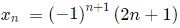So x49  = 99 and x50 = −101
Therefore x49 + x50  = −2

Q.5. For a real number x the condition ∣3x−20∣+∣3x−40∣ = 20 necessarily holds if       
(a)
10 < x < 15
(b) 9 < x < 14
(c) 7 < x < 12
(d) 6 < x < 11

Correct Option is C
Case 1 : x ≥ 40 / 3
we get 3x - 20  + 3x - 40 = 20
6x = 80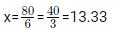Case 2: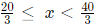we get 3x - 20 + 40 - 3x = 20
we get 20 = 20
So, we get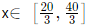Case 3: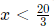we get 20 - 3x + 40 - 3x = 20
40 = 6x
x = 20/3
but this is not possible
so we get from case 1,2 and 3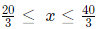Now looking at options
we can say only option C satisfies for all x .
Hence 7 < x < 12.

Q.6. If n is a positive integer such that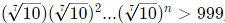, then the smallest value of n is       

Ans: 6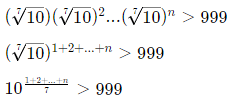For minimum value of n,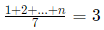1 + 2 + ... + n = 21
We can see that if n = 6, 1 + 2 + 3 + ... + 6 = 21.

Q.7. If 3x + 2∣y∣ + y=7 and x + ∣x∣ + 3y = 1 then x + 2yx + 2y is:       
(a)
-4/3
(b) 8/3
(c) 0
(d) 1

Correct Option is C
We need to check for all regions:
x >= 0, y >= 0
x >= 0, y < 0
x < 0, y >= 0
x < 0, y < 0
However, once we find out the answer for any one of the regions, we do not need to calculate for other regions since the options suggest that there will be a single answer.
3x + 3y = 7
2x + 3y = 1
Hence, x = 6 and y = -11/3
Since y > = 0, this is not satisfying the set of rules.
Next, let us test x >= 0, y < 0,
3x - y = 7
2x + 3y = 1
Hence, y = -1
x = 2.
This satisfies both the conditions. Hence, this is the correct point.
We need the value of x + 2y
x + 2y = 2 + 2(-1) = 2 - 2 = 0.

Q.8. If a, b and c are positive integers such that ab = 432, bc = 96 and c < 9, then the smallest possible value of a + b + c is     
(a) 56
(b) 59
(c) 49
(d) 46

Correct Option is D
Given ab = 432, bc = 96 and c < 9
To find the minimum value for a + b + c, the two larger numbers should be as close as possible.
The closest combination whose product is 432 is 18 x 24 . For b = 24, we get c = 4 and a = 18 .
Hence the least value for a + b + c = 46 .

Q.9. How many 3-digit numbers are there, for which the product of their digits is more than 2 but less than 7?      

Ans: 21
The product of the digits of the three-digit numbers should be more than 2 and less than 7 .
Hence the possible numbers are as follows.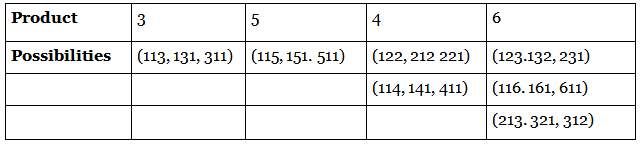Q.10. The mean of all 4 -digit even natural numbers of the form 'aabb', where a >0, is       
(a) 5050
(b) 4466
(c) 5544
(d) 4864

Correct Option is C
The sum of possible even digit numbers in the form aabb is 1100 + 1122 + 1144 + 1166 + 1188 + 2200 + 2222 + 2288 + ......9900 + 9922 + 9988 i.e. (45 numbers)
⇒ 1100 + 1100 +1100+ 1100 + 1100 + 22 + 44 + 66 + 88 + 2200 + 2200 + 2200 + 2200 + 2200 +22 + 44 + 66 + 88 +… + 9900 + 9900 + 9900 + 990 + 9900 + 22 + 44 + 66 + 88
⇒ 5(1100 + 2200 + .....9900) + 9(22 + 44 + 66 + 88)5 x 1100(1 + 2 + .... 9) + 9 x  22(1 + 2 + 3 + 4)
⇒ 5500(45) + 45 ´ 44 = 45(5544)
Hence mean =5544

Q.11. Let A, B and C be three positive integers such that the sum of A and the mean of B and C is 5. In addition, the sum of B and the mean of A and C is 7. Then the sum of A and B is      
(a) 6
(b) 5
(c) 7
(d) 4

Correct Option is A
Given A, B and C are positive integers such that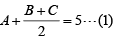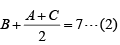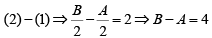The least value for A=1 in which case B=5.
Hence A + B = 6

Q.12. If x and y are positive real numbers satisfying x + y=102, then the minimum possible value of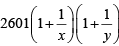is      

Ans: 2704
AM ≥ GM ≥ HM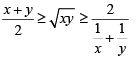Given x + y = 102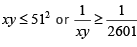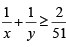The minimum value of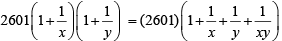=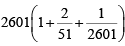= 2704

Q.13. How many 4-digit numbers, each greater than 1000 and each having all four digits distinct, are there with 7 coming before 3?      

Ans: 315
Consider four blanks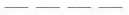7 is in thousand place, then 3 can be placed in any of the 3 places in 3 ways. Remaining two blanks can be filled with remaining eight digits in 8P2 ways. The number of numbers that have 7 is in thousand place is 3  x 8P2 = 168
Thousand place cannot be 0,7 and 3, it can be filled with remaining 7 digits in 7 ways. In remaining 3 blanks, 7 and 3 can be arranged in 3 ways. Fourth blank can be filled in 7 ways. The number of numbers that are formed where 7 and 3 is not in thousand place is 7 x 3 x 7 = 147 . Hence total required numbers = 168 + 147 = 315 .

Q.14. In how many ways can a pair of integers (x , a) be chosen such that x2 - 2| x| + | a - 2| = 0?      
(a) 4
(b) 5
(c) 6
(d) 7

Correct Option is D
x2 - 2| x| + | a- 2 |= 0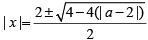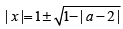If a > 2; | a - 2 |= a - 2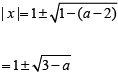since x is integer 3 - a ≥ 0
a ≤ 3
The possible values of a is = 3
Then x = ± 1;
If a = 2, | x |=| 1 ± 1 |, ⇒ x = ± 2, 0
If a < 2, | a - 2 |= 2 - a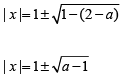Since x is integer a -1 ≥ 0 ⇒ a ≥ 1
∴ The possible values of a is 1
If a = 1, | x |= 1 ⇒ x = ± 1
∴ The possible pairs =(-1,3), (1,3), (1,1), (-1,1), (2,2),(-2,2), (0,2) i.e., 7

Q.15. The number of integers that satisfy the equality ( x2 - 5x + 7 ) x+11 is      
(a) 2
(b) 3
(c) 5
(d) 4

Correct Option is B
( x2 - 5x + 7 ) x+1  = 1
We know, for ab = 1,
if -a = -1 then b is even.
-a = 1 then b is any number
-a > 0 then b = 0
Case 1: x + 1 = 0 Þ x = -1
Case 2: x2 -5x+7 =1 ⇒ x2 -5x + 6 = 0 ⇒ x = 2 or 3
Case 3: x-5x + 7 = -1 ⇒ x2 -5x + 8 = 0
but x is not an integer
∴ The number of integers satisfies the equation is 3

Q.16. The number of pairs of integers (x , y) satisfying x ≥ y ≥ -20 and 2 x + 5 y = 99 is      

Ans: 17
2 x + 5 y = 99
When y = - 19, x = 97; since x ≥ y ; the maximum value of y is 13 and corresponding value of x is 17.
We know that the solutions of y are in arithmetic progression with common difference 2.
Here a = - 19, d = 2, tn = 13
tn = a + (n -1)d
-19 + (n - 1)(2) = 13
(n - 1)2 = 32 ⇒ n = 17
Hence number of pairs integers is 17

Q.17. If x and y are non-negative integers such that x + 9 = z, y + 1 = z and x + y < z + 5, then the maximum possible value of 2x + y equals      

Ans: 23
Given x + 9 = z = y + 1 and x + y < z + 5
⇒ (z -9) + (z - 1) < z + 5
⇒ z < 15
Hence the maximum value of z = 14, max of x = 5 and max of y = 13
Required answer, 2 x + y = 2 x 5 + 13 = 23

Q.18. How many pairs (a, b) of positive integers are there such that a ≤ b and ab = 42017 ?      
(a) 2017
(b) 2019
(c) 2020
(d) 2018

Correct Option is B
Given, a × b = 42017 = 24034
Since a × b = 42017 , is a perfect square the number of factors of 24034 is odd.
Required answer, the number of values of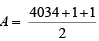= 2018

Q.19. If x1 = -1 and xm = xm +1 + (m + 1) for every positive integer \$m,\$ then x100 equals       
(a) -5151
(b) -5150
(c) -5051
(d) -5050

Correct Option is D
xm + 1 = xm - (m + 1)
x= x- 2 = -1 - 2 = -3
x3 = x2 - 3 = -1 - 2 - 3 = - 6
Similarly,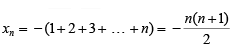Hence,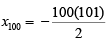= -5050

Q.20. Let m and n be natural numbers such that n is even and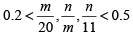. Then m - 2n equals      
(a) 3
(b) 4
(c) 1
(d) 2

Correct Option is C
Given,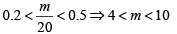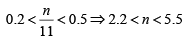⇒ n = 4
since 0.2 < n/m < 0.5 m and n = 4, m = 9
m - 2n = 9 - 2 x 4 = 1

Q.21. Let N, x and y be positive integers such that N = x + y, 2 < x < 10 and 14 < y < 23. If N > 25, then how many distinct values are possible for N?      

Ans: 6
Given, 2 < x < 10 and 14 < y < 23 Þ 17 < ( x + y ) < 32 i.e. 17 < N < 32
But N > 25 hence 25 < N < 32
N can take 6 distinct values.

Q.22. How many of the integers 1, 2, … , 120, are divisible by none of 2, 5 and 7?      
(a) 40
(b) 42
(c) 43
(d) 41

Correct Option is D
=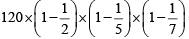= 41.14
Required answer is the integral part of 41.14 = 41

The document CAT Previous Year Questions : Number System - Notes | Study Quantitative Aptitude (Quant) - CAT is a part of the CAT Course Quantitative Aptitude (Quant).
All you need of CAT at this link: CAT

## Quantitative Aptitude (Quant)

163 videos|163 docs|131 tests
 Use Code STAYHOME200 and get INR 200 additional OFF

## Quantitative Aptitude (Quant)

163 videos|163 docs|131 tests

### How to Prepare for CAT

Read our guide to prepare for CAT which is created by Toppers & the best Teachers

Track your progress, build streaks, highlight & save important lessons and more!

,

,

,

,

,

,

,

,

,

,

,

,

,

,

,

,

,

,

,

,

,

;